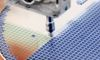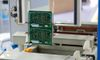# Mastering PID Tuning: The Comprehensive Guide11 Jul, 2023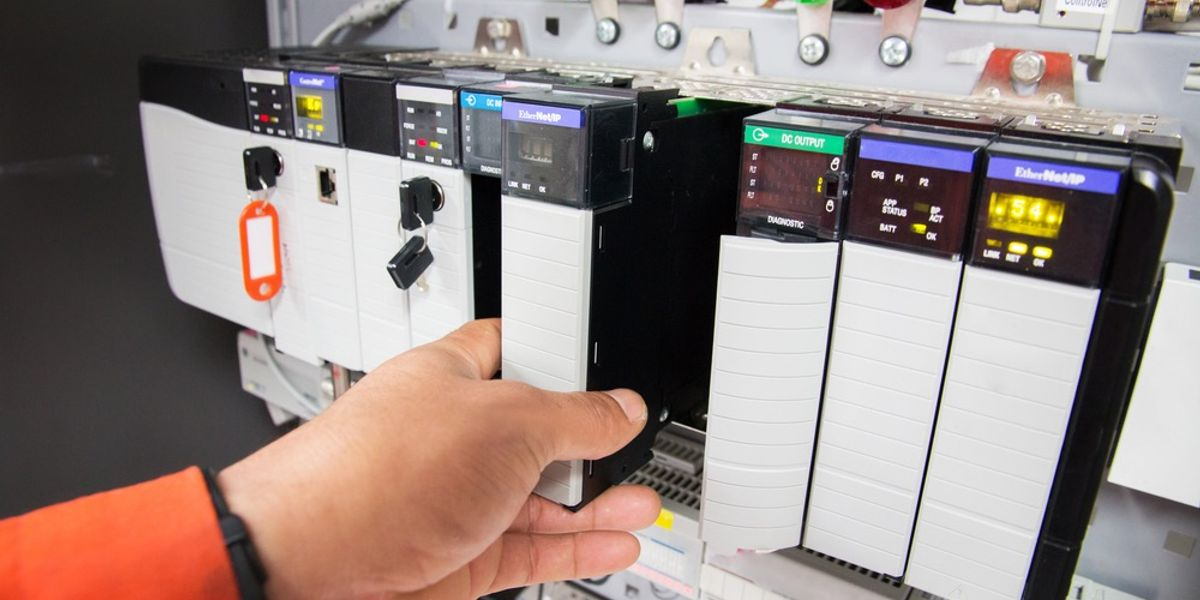Achieving precision in PID tuning yields remarkable outcomes, such as increased system efficiency, responsiveness, and overall operational performance

## 1. Introduction

PID tuning represents a pivotal process in control systems, dictating the performance and stability of systems across various industries - from HVAC control to drone navigation. A PID (Proportional, Integral, Derivative) controller's responsibility lies in minimizing error by adjusting a control variable, such as the throttle of an engine or a heater's power level. The art of PID tuning hinges on setting optimal values for the proportional, integral, and derivative constants, making the controller respond to the system's dynamic changes. In this comprehensive guide, we will explore the concept of PID tuning, its relevant terms, the process and real life applications. By the end, you will gain a solid understanding of PID tuning and be equipped with the knowledge to apply it effectively in your control system endeavors.

## 2. Understanding PID Control

Central to various automated systems, PID control stands for Proportional, Integral, Derivative control. It's a type of feedback controller, manipulating an input to a system to get the desired output. The mechanism involves monitoring the error signal, which is the difference between a setpoint and the measured variable. These components work in harmony to minimize error and optimize the control variable, allowing the system to achieve and maintain the desired setpoint.

The PID controller's functionality revolves around three distinct parameters - Proportional, Integral, and Derivative. These parameters shape the controller output to drive the system towards the desired state. Each component corresponds to present, past, and future error values, providing a comprehensive control strategy.

Understanding each term's workings in isolation is crucial to grasp the overall PID control mechanism. So, let's delve into the specifics, starting with the Proportional term.

### 2.1. The Proportional Term

The Proportional term in a PID controller, often signified by the gain Kp, directly influences the control effort in proportion to the error signal. If we denote the error as e(t), the control loop output from the proportional term can be represented as Kp*e(t).

Consider a thermostat controlling room temperature. If the current room temperature deviates significantly from the setpoint, the error will be large. A high Kp value in this situation would result in a considerable change in heating or cooling power. Thus, the proportional control's response is immediate but depends on the error magnitude.

The balance is essential here; a small Kp may result in a sluggish system response, taking longer to reach the setpoint. In contrast, an excessively large Kp can cause a rapid response leading to overshoot and system instability. Therefore, finding an optimal Kp value is crucial to system performance. It's here where the art and science of PID tuning come into play, setting the foundation for a system's responsiveness.

### 2.2. The Integral Term

The Integral component, denoted by Ki, accumulates the error over time and, like the Proportional term, scales it. It has a significant role in eliminating the steady-state error, a constant difference that persists between the desired and actual system output even after the initial system response.

Mathematically, the integral term is represented as Ki ∫e(t)dt, where e(t) is the error at time t, Ki is the integral gain, and the integral symbol denotes the accumulation of the error over time. This integral sum continually increases as long as the error is present, driving the control output to minimize the error.

Let's revisit our thermostat example. Assume that the room temperature has stabilized at a value slightly below the setpoint. Despite the proportional component's efforts, a small but consistent error persists - this is the steady-state error. The integral component, accumulating this error over time, amplifies the control output, nudging the system towards the setpoint.

The integral term can be both a savior and a challenge in PID control. While it is effective in eliminating steady-state errors, it can also introduce instability or oscillations due to excessive control loop action, especially in systems with slow dynamics. The integral component keeps accumulating error and can wind-up to large values during a large or prolonged error. This wind-up can lead to a significant overshoot when the error reduces. Hence, careful tuning of the integral gain, Ki, is crucial to balance system performance and stability.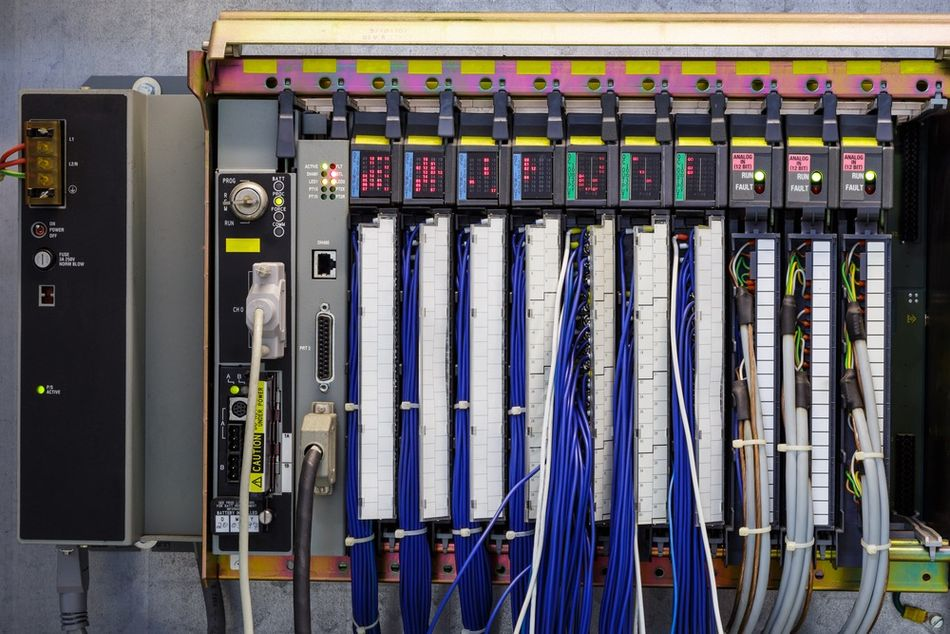The PID controller's functionality revolves around three distinct parameters - Proportional, Integral, and Derivative

### 2.3. The Derivative Term

The Derivative term in a PID controller, denoted by Kd, responds to the rate of change of the error. This component brings a predictive aspect to the PID control strategy, allowing the controller to act before a significant error accumulates.

Formally, the derivative control output can be represented as Kd * de(t)/dt, where de(t)/dt is the rate of change of the error at time t, and Kd is the derivative gain. As the derivative of the error, this term responds most strongly when the error is changing rapidly, thereby preventing large swings and reducing overshoot.

Returning to the thermostat example, let's imagine the room temperature is rapidly rising towards the setpoint due to a significant increase in heating power. The derivative action component, sensing this quick change, will start decreasing the control loop output even before the setpoint is reached. This dampens the system response and prevents a potential overshoot.

However, implementing derivative action control requires careful consideration. A high derivative gain can make the system sensitive to noise in the error signal, as the derivative term will amplify any rapid fluctuations. Moreover, in a system where the error changes slowly or not at all, the derivative term will be zero, making it ineffective in such situations.

Therefore, when tuning a PID controller, it's essential to balance the effects of all three terms to ensure a stable, fast, and accurate system response.

Recommended Reading: PID Loops: A Comprehensive Guide to Understanding and Implementation

## 3. The Process of PID Tuning

PID tuning is an iterative process that adjusts the controller tuning parameters to optimize the system's response. It involves modifying the gains of the proportional, integral, and derivative terms to achieve the desired system behavior. Depending on the specific application and system, the desired behavior might be fast response, minimal overshoot, or even steady-state accuracy.

PID tuning is a systematic process aimed at finding the optimal values for the proportional, integral, and derivative parameters of a PID controller tuning. The objective is to fine-tune these parameters to achieve the desired control system performance, including fast response, minimal overshoot, and robust stability. The process of PID tuning typically involves several steps.

First, an initial set of parameters is selected based on prior knowledge or best practices. Then, the system's response to changes in setpoint or disturbances is observed and analyzed. If the response is not satisfactory, adjustments are made to the PID parameters.

There are various methods and techniques available for PID tuning, including manual tuning, Ziegler-Nichols method, and model-based approaches. These methods involve iteratively adjusting the parameters, observing the system's response, and iteratively refining the parameters until the desired performance is achieved.

Three primary methods are used in PID tuning: auto-tuning, manual tuning, software-based tuning, and formal mathematical methods. Each has its advantages and applications, and the choice depends on the system complexity, the required accuracy, and the availability of system models and computing resources.

### 3.1. Manual PID Tuning

Manual PID tuning involves adjusting the controller gains based on the observed system response and the tuner's experience and knowledge. This method is often used when a mathematical model of the system is not available, or the system dynamics are too complex for analytical methods.

The typical process for manual tuning starts with setting all gains to zero. Then, the proportional gain (Kp) is slowly increased until the system begins to oscillate. This oscillation point indicates that the system is on the verge of instability, and the proportional gain at this point is recorded as Kp_critical.

Next, the integral (Ki) and derivative (Kd) gains are introduced. The integral gain is increased until any steady-state error is eliminated, while ensuring the system doesn't become unstable. Similarly, the derivative gain is adjusted to prevent overshoot and reduce the settling time.

It's important to note that manual tuning requires a delicate balance and a deep understanding of the system. It may not be suitable for systems with nonlinear dynamics or where high precision is required.

### 3.2. Automated PID Tuning

Automated PID tuning, as the name suggests, is a process where the tuning of the PID controller parameters is done automatically using software algorithms. These methods are more precise and less labor-intensive than manual tuning and are preferred when a mathematical model of the system is available or can be derived.

Auto-tuning techniques use the system's response to a specific input, such as a step or impulse, to estimate the system's parameters. These parameters are then used to calculate the optimal PID gains. Various algorithms can be used for automated PID tuning, including the Ziegler-Nichols method, the Cohen-Coon method, and various optimization algorithms.

The Ziegler-Nichols method is one of the most commonly used automated PID tuning methods. The method consists of two steps: first, the critical gain and critical period of the system are determined, and then these values are used to calculate the PID gains using empirically derived formulas. Although the Ziegler-Nichols method can provide a good starting point, it tends to result in aggressive control action, leading to a system response with substantial overshoot.

Another automated tuning method is the Cohen-Coon method, which is primarily used for processes with long time delays. This method involves applying a step change to the system and observing the system's response. From this response, the controller's process reaction curve is derived, which is used to calculate the PID gains.

Optimization algorithms such as genetic algorithms, particle swarm optimization, and simulated annealing can also be used for automated PID tuning. These methods treat the tuning process as an optimization problem, where the objective is to find the set of PID gains that minimize a certain performance index. This index is usually a measure of the system's transient and steady-state errors.

It should be noted that each auto-tuning method has its strengths and weaknesses, and the best method depends on the specifics of the system and application. Automated tuning methods also require a higher level of expertise to implement and interpret, compared to manual tuning.

Recommended Reading: Fine-tuning device performance with swarms of swimming cells

## 4. Advanced PID Tuning Techniques

As one gains expertise and deeper understanding of the PID control system, advanced tuning techniques may be employed to enhance system performance. These techniques offer an improved level of control, helping to minimize system errors and optimize efficiency.

One such technique is the implementation of gain scheduling. Gain scheduling is a method in which PID parameters are adjusted in real-time based on the current state of the system. This approach is especially useful in non-linear systems where system dynamics change with operating conditions. For example, an unmanned aerial vehicle (UAV) adjusting its PID gains for altitude control depending on whether it's ascending, hovering, or descending.

Adaptive control is another advanced tuning method. It differs from gain scheduling in that it can adjust controller tuning parameters on the fly without needing predefined states or conditions. The adaptive controller uses real-time data and a control algorithm to adjust the PID parameters as the system operates. This technique is powerful but also more complex to implement, and it requires a robust model of the system to be controlled.

Yet another advanced technique is the use of robust control strategies. Robust control aims to achieve good performance over a wide range of system conditions and is particularly useful in systems with uncertain parameters or external disturbances. The H-infinity method is a common robust control technique used for PID controllers.

In model predictive control (MPC), a model of the system is used to predict the future output. The PID parameters are then adjusted to minimize the difference between the predicted output and the desired output over a certain future time horizon. The advantage of MPC is that it can handle multi-input, multi-output systems and constraints on the system inputs and outputs. However, the implementation of MPC requires significant computational resources and a good model of the system.

Lastly, the optimal control technique seeks to optimize a certain performance index, such as energy consumption or error minimization. This technique requires the formulation of a cost function that quantifies the performance index and the use of optimization algorithms to find the PID parameters that minimize this cost function.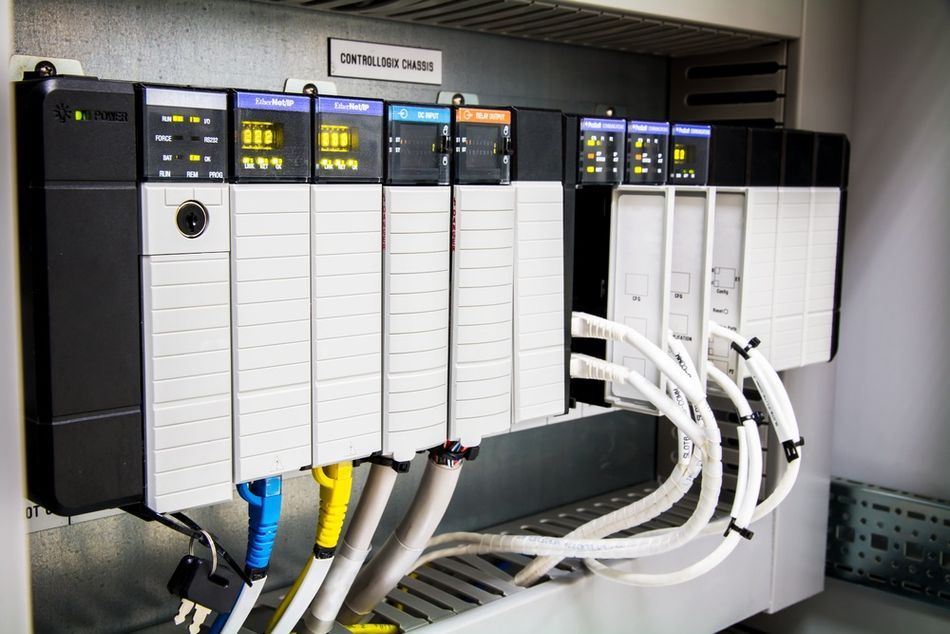Automated tuning methods also require a higher level of expertise to implement and interpret, compared to manual tuning

## 5. Application Examples of PID Tuning

PID controllers find extensive use in various industrial processes, robotics, and even everyday electronics. The principles of PID tuning are applicable across a diverse range of systems, adjusting to specific needs and requirements. Here are a few illustrative examples.

Cruise Control in Automobiles: A modern automobile uses a PID controller for its cruise control system. The desired speed set by the driver is the setpoint. The car's current speed, read from the speedometer, is the process variable. The PID controller manipulates the throttle position to maintain the set speed. Advanced tuning techniques are applied to account for varying conditions such as road gradient or load changes (like climbing a hill or overtaking).

Temperature Control in Industrial Processes: Many industrial processes require maintaining a specific temperature. For example, in a chemical reactor, maintaining temperature within a specified range is critical for reaction efficiency and safety. A PID controller uses temperature readings from a sensor as the process variable and manipulates the heating element to maintain the desired temperature setpoint. Over time, the system might need tuning to respond to changes such as wear on the heating element or variations in ambient temperature.

Drone Flight Stabilization: Drones rely on PID controllers for stabilizing their flight. The drone's inertial measurement unit provides data on its current orientation (the process variable), which the PID controller compares to the desired orientation (the setpoint). The controller then adjusts the speed of the drone's motors to correct any deviation. Tuning the PID controller in a drone is a complex task due to the non-linear nature of the system and the presence of external disturbances such as wind.

Industrial Robotics: PID controllers are extensively used in industrial robotics, for example, to control the position of a robotic arm. The controller takes the current position of the arm as the process variable and compares it with the desired position (setpoint). The controller then manipulates the motor torques to move the arm to the desired position. PID tuning in this context can be challenging due to the multi-joint nature of robotic arms and the need for precise positioning.

In each of these applications, PID tuning is essential to ensure efficient, accurate, and safe operation of the system. Advanced tuning techniques, including those discussed earlier, can be used to enhance system performance under varying operating conditions and requirements.

## 6. Common Pitfalls and How to Avoid Them

PID tuning is often considered more of an art than a science, mainly because of the trial and error involved and the need for expertise to understand how each term interacts with the others. A wide range of pitfalls can occur during the PID tuning process. By understanding these potential pitfalls, you can effectively avoid them or mitigate their impacts.

Tuning without Understanding the System: One of the most common mistakes in PID tuning is not fully understanding the dynamics of the system being controlled. Different systems will respond differently to changes in the PID controller's settings, and without a solid grasp of these dynamics, effective tuning can be challenging. Therefore, before starting the tuning process, it is advisable to perform a thorough analysis of the system, identifying its dynamics, response times, and stability conditions.

Over-Reliance on the Proportional Term: It's a common misstep to over-rely on the proportional term to do the heavy lifting. Over-tuning the proportional term can lead to large oscillations and instability. A high proportional gain can cause the system to react aggressively to errors, leading to overshoot and oscillation about the setpoint. Balancing the use of all three terms – proportional, integral, and derivative – is crucial for stability and optimum performance.

Ignoring the Derivative Term: The derivative term is often misunderstood or ignored due to its dependence on the rate of change of the error. It can introduce noise amplification in the presence of measurement noise, leading many to avoid using it. However, a well-tuned derivative term can improve the transient response and stability of the system, reducing overshoot and settling time. Proper filtering techniques can be used to mitigate the effects of noise.

Neglecting Controller Saturation: Controller saturation occurs when the controller output exceeds its maximum or minimum limit. This often happens when high gain values are used, or when a large disturbance pushes the controller beyond its limits. Saturation can cause integral windup, where the integral term accumulates a large error during the saturation period and causes an overshoot when the controller comes out of saturation. Anti-windup strategies, such as clamping or back calculation, can be employed to handle integral windup.

Not Accounting for Nonlinearities: PID controllers are based on linear control theory, but many real-world systems exhibit non-linear behavior. For such systems, PID controllers may not perform optimally across the full operating range. Gain scheduling is a technique commonly used to overcome this problem, where different sets of PID parameters are used at different operating points.

## 7. Conclusion

PID tuning is a pivotal process in control engineering, and its role can't be overemphasized in creating efficient and responsive systems. While it might seem daunting due to the complexity and interaction between the proportional, integral, and derivative terms, a structured approach to tuning, as outlined, makes it manageable.

A solid understanding of the control system is the foundation for successful PID tuning. With this understanding, an engineer can then methodically adjust the controller tuning parameters, observing system behavior and making incremental changes until an optimal response is achieved. Advanced tuning techniques, such as gain scheduling and anti-windup strategies, add layers of sophistication to cope with non-linear behavior and controller saturation.

The lessons learned from real-world applications of PID tuning provide invaluable insights for addressing similar challenges. From maintaining a steady temperature in a furnace to achieving stable altitude control in a drone, the principles of PID tuning find universal application.

Awareness of common pitfalls and their solutions is another cornerstone for effective PID tuning. Avoiding mistakes such as over-reliance on the proportional term, ignoring the derivative term, neglecting controller saturation, and not accounting for non-linearities can significantly improve the PID tuning process.

## 8. Frequently Asked Questions (FAQs)

Q. What is PID Tuning?

PID tuning is the process of finding the optimal parameters (proportional, integral, and derivative gains) for a PID controller to achieve desired system behavior.

Q. Why is PID Tuning important?

PID tuning is critical in control systems to ensure that the system responds quickly and accurately to changes in the input or disturbances. Improperly tuned systems can be slow to respond, overshoot their target, or even become unstable.

Q. What are the steps in PID tuning?

The steps involve understanding the system dynamics, starting with default or small PID values, gradually increasing proportional gain until the system starts to oscillate, then adjusting the integral gain to reduce steady-state error, and finally tuning the derivative gain to dampen overshoot.

Q. What is controller saturation and how can it be avoided?

Controller saturation occurs when the PID controller output exceeds its maximum or minimum limit. This can be avoided by using anti-windup strategies, such as clamping or back calculation, which help to handle the accumulation of error (windup) in the integral term during saturation.

Q. What is gain scheduling?

Gain scheduling is a method used for controlling non-linear systems, where different sets of PID parameters are used at different operating points. It helps overcome the limitations of PID controllers when dealing with non-linear behavior across the full operating range.

## References:

https://realpars.com/pid-tuning/

https://www.mathworks.com/discovery/pid-tuning.html

https://www.omega.co.uk/prodinfo/how-to-tune-a-pid-controller.html

https://www.incatools.com/pid-tuning/pid-tuning-methods/